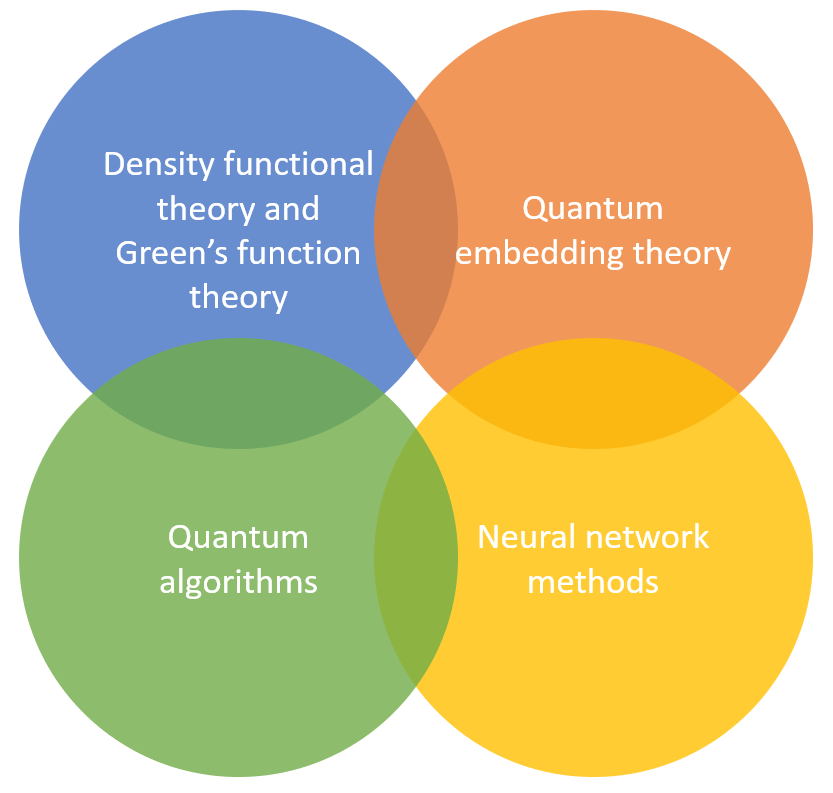So you take a picture of something you see
In the future where will I be?
You can climb a ladder up to the sun
Or write a song nobody has sung
Or do something that's never been done
Do something that's never been done

--Coldplay, "Talk"

My interest

My main interest is to develop efficient, accurate and scalable numerical algorithms and mathematical software tools for ground state and excited state electronic structure theories. I find this field particularly exciting because
1. Scientifically, it is amazing that many (electronic, mechanical, optical) properties calculated from such first principle theories (i.e. the only input is atomic specicies and atomic positions. There is in principle no dependence on empirical or fitting parameters) can be directly compared to experimental results. Electronic structure theory has also been identified as one of the potential "killer apps" for quantum computation.
2. Mathematically, the theories are relatively well defined. They also provide mathematical and computational challenges not commonly seen in other branches of applied mathematics. The complexity of electronic structure theories can range from high (like density functional theory) to very high (like configuration interaction), and hence numerical methods with reduced complexity are urgently needed in order to simulate ever larger relastic systems.
3. This field had been active in physics and chemistry before computers even existed, and yet the field is still at its early stage from the perspective of applied mathematics. In my view, "language barrier" is sometimes the biggest obstacle preventing mathematicians from understanding the problem. There is great potential for tools in numerical analysis and numerical linear algebra to deeply impact this field.
While continuing the development of large scale DFT and TDDFT based methods, recently I am particularly interested in numerical algorithms for strongly correlated quantum systems, using 1) quantum computers 2) quantum embedding theory 3) neural networks.
The picture below summarizes my current research interest:Introductory material

In Summer 2016, with Jianfeng Lu, we developed a summer school course, aimed at introducing electronic structure theory specifically to students and researchers with mathematical backgrounds. In particular, it does not assume that you already know quantum mechanics (we start from scratch and then move very quickly).

The video of the lectures is available on the MSRI website (2.5 hours each day for 10 days).

In Fall 2018, I gave a topic course (Math 275): Mathematical introduction to electronic structure Theory. All course materials, including lecture notes, notebooks (mostly in Julia), and assignments are on the Github page.

Based on such materials, we wrote an introductory level textbook. This book provides a self-contained, mathematically oriented introduction to the subject and its associated algorithms and analysis. It aims at helping applied mathematics students and researchers with minimal background in physics understand the basics of electronic structure theory, and be prepared to conduct research in this area.

A Mathematical Introduction to Electronic Structure Theory (SIAM book)

In Fall 2021, I gave a topic course (Math 275): Quantum Algorithms for Scientific Computation. It focused on quantum algorithms closely related to scientific computation, and in particular, matrix computation.

Lecture notes on Quantum Algorithms for Scientific Computation